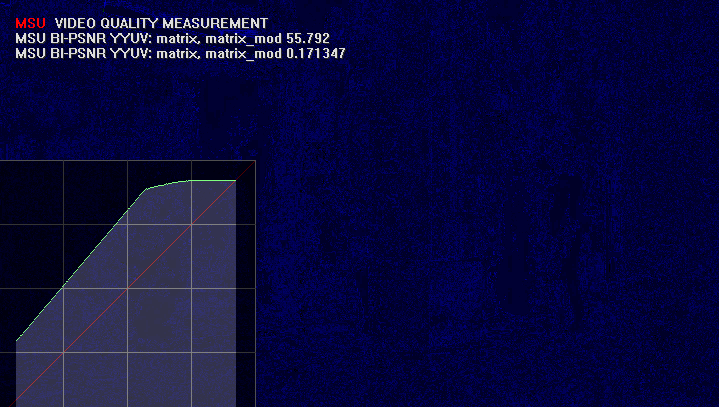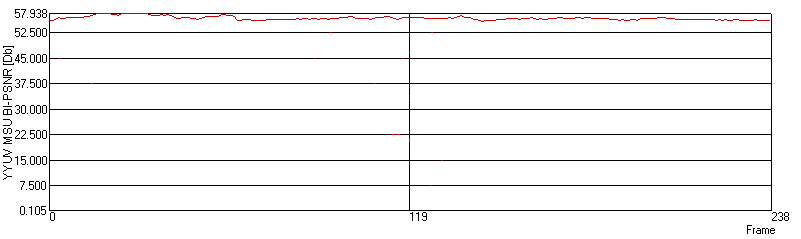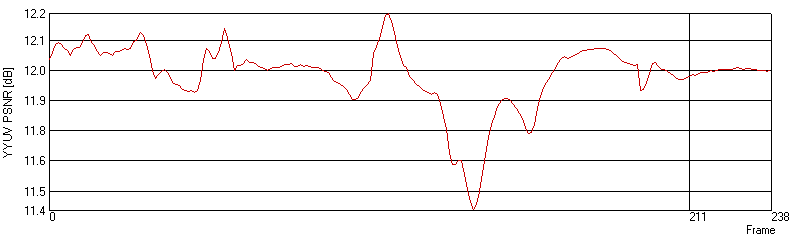# MSU Brightness Independent PSNR (BI-PSNR)

• Project, Ideas: Dr. Dmitriy Vatolin
• Implementation: Alexey Noskov
• Updating and additions: Sergey Grishin

## Common idea

BI-PSNR metric is intended for measuring distortions in video taking into account brightness shifts.

## Change Log

[!] — Known bug
[+] — New Feature
[*] — Other

Version 1.1
[*] Visualization bug fixed for non-stadard resolution video

Version 1.0
[+] First plugin release

## Usage

BI-PSNR metric should be used when one of the sequences has any brightness transformation, which does not change within frame. Example of such transformation is uniform increasing of brightness of contrast for single frame of for all sequence. Such transformations prevent usage of standard metrics because of strong brightness difference between comparing frames. BI-PSNR algorithm calculates brightness transformation, which makes frames similar as possible and calculates standard PSNR and MSE metrics taking into account founded transformation.

## Visualization

There are two part of visualization:

• MSE visualization for frame. Colors of visualization are standard for MSE (in order of error increasing): black-blue-green-red.
• Brightness transformation plot. X-axis is brightness at the first sequence, Y-axis - brightness on the second one. Green points are values, which corresponds to each other (brightness transformation). Red diagonal is identical transformation (no brightness changes).Example of visualizationVisualization of the same frame using standard PSNR

## Plots

Plots of per-frame PSNR values after the found transformation are drawing after all calculations. Plots are entirely the same as standard per-frame PSNRPlot's exampleStandard PSNR plot for the same sequence

## Algorithm

Table C[i,j] is filling for each frame: C[i,j] = { number of points in the same position, which have brightness i at the first sequence frame and j at the second sequence frame }

Next, for each i (brightness value from the first frame) we find corresponding brightness from the second sequence. Following formula is used to estimate distanse from arbitrary values of i and j:One can note that this formula is sum of quadratic differences between all pixels of the first sequence with value i and all corresponding pixels from second sequence on the assumption that brightness was shifted to i-j.When transformation was found, we can find MSE for the frame taking into account this transformation: